# Volume Formula

Here we will learn about volume formulas, including the formula for calculating the volume of 3D shapes and how to use the volume formula to solve problems.

There are also worksheets based on Edexcel, AQA and OCR exam questions, along with further guidance on where to go next if you’re still stuck.

## What is a volume formula?

A volume formula is a formula which is used to calculate the volume of a 3D shape. Volume is the amount of space there is inside a shape.

To calculate the volume of an object in three dimensions, we can use the various volume formulas.

Cube
\text { Volume }=a^3
Cuboid
(or rectangular prism)

\text{Volume}=l\times w\times h
Prism
\text{Volume}=\text{Area of cross section} \times \text{Length}
Cylinder
\text{Volume}=\pi r^2h
Pyramid
\text{Volume}=\frac{1}{3}\times \text{Area of base} \times \text{Height}
Cone
\text{Volume}=\frac{1}{3}\pi r^2h

(\text{Volume}=\frac{1}{3}\times \text{Area of base} \times \text{Height})
Sphere
\text{Volume}=\frac{4}{3}\pi r^3

The formulae for the volume of a cone and the volume of a sphere are given to you for GCSE maths. You need to learn the other formulae.

Volume is measured in cubic units.

• Metric units examples

cm^3 (cubic centimetres),

m^3 (cubic metres),

mm^3 (cubic millimetres).

• Imperial units examples

cubic feet,

cubic inches.

Volume can also be described using units of capacity such as millilitres, litres, pints or gallons.

### What is a volume formula?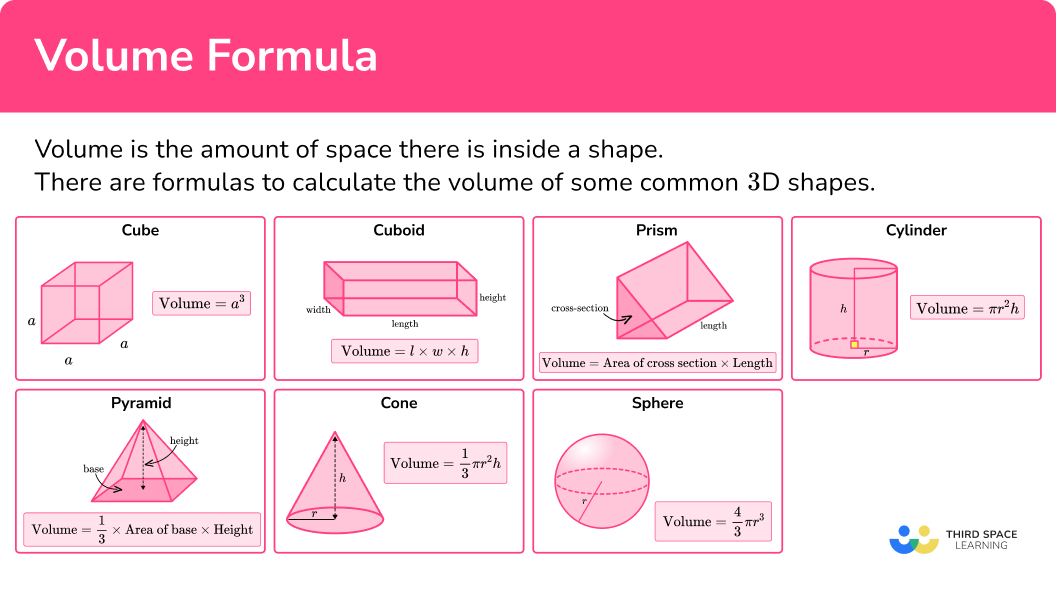## How to use volume formula

In order to use volume formula:

1. Write down the formula.
2. Substitute the values into the formula.
3. Complete the calculation.
4. Write the answer, including the units.

### Explain how to use volume formula### Related lessons on how to calculate volume

Volume formula is part of our series of lessons to support revision on how to calculate volume and 3D shapes. You may find it helpful to start with the main 3D shapes lesson for a summary of what to expect, or use the step by step guides below for further detail on individual topics. Other lessons in this series include:

## Volume formula examples

### Example 1: volume of a cylinder

Calculate the volume of the cylinder below.

1. Write down the formula.

The 3D shape is a cylinder. The formula you need to use is

\text{Volume}=\pi r^2h.

2Substitute the values into the formula.

We need to calculate the area of a circle (the circular base) and then multiply it by the height of the shape.

The values you need to substitute into the formula are the radius of the base (r=5) and the height of the shape (h=13).

\begin{aligned} \text{Volume}&=\pi r^2h \\\\ &=\pi \times 5^2 \times 13 \end{aligned}

3Complete the calculation.

V=325\pi=1021.107…

4Write the answer, including the units.

The dimensions of the cylinder were given in centimetres, so the volume (the amount of three-dimensional space inside the shape) will be in cubic centimetres (cm^3).

V=1021 \ cm^3 (to the nearest integer).

### Example 2: volume of a sphere

Calculate the volume of a sphere with diameter 9 \ mm.

The 3D shape is a sphere. The formula you need to use is

\text{Volume}=\frac{4}{3}\pi r^3.

You need to substitute the value of the radius of the sphere into the formula. You need to divide the diameter by 2 to calculate the radius r.

r=9\div 2=4.5

\begin{aligned} \text{Volume}&=\frac{4}{3} \pi r^3 \\\\ &=\frac {4}{3}\times \pi \times 4.5^3 \end{aligned}

V=\frac{243}{2}\pi=381.703…

The dimensions of the sphere were given in millimetres, so the volume of the sphere will be in cubic millimetres (mm^3).

V=382 \ mm^3 \ (to 3 significant figures).

### Example 3: missing dimension

The volume of the cuboid is 2016 \ cm^{3}.

Calculate the value of x.

The 3D shape is a cuboid. The formula you need to use is

\text{Volume}=l\times w\times h.

This formula is very similar to the formula for the volume of a cube.

The values you need to substitute into the formula are \text{Volume}=2016, l=8 and w=14.

For the height (h) you can use x.

The length (l), the width (w) and the height (h) of the cuboid are interchangeable, so it doesn’t matter which dimension l or w or h.

\begin{aligned} \text{Volume}&=l\times w\times h \\\\ 2016&=8\times \times 14\times x\\\\ 2016&=112\times x \end{aligned}

x=2016\div 112=18

The dimensions of the cuboid were given in centimetres and the volume was given in cubic centimetres (cm^3).

x=18 \ cm

### Example 4: missing dimension

The volume of the square pyramid is cuboid is 66 \ 000 \ cm^{3}.

Calculate the height of the pyramid.

The 3D shape is a pyramid. The formula you need to use is

\text{Volume}=\frac{1}{3}\times \text{area of base} \times \text{height}.

First you need to work out the area of the square base, 60^2=60\times 60=3600.

The values you need to substitute into the formula are \text{volume}=66 \ 000 and \text{area of base}=3600.

For the height you can use (h).

\begin{aligned} \text{Volume}&=\frac{1}{3}\times \text{area of base} \times \text{height}\\\\ 66 \ 000&=\frac {1}{3}\times 3600\times h\\\\ 66 \ 000&=1200\times h \end{aligned}

h=66 \ 000\div 1200=55.

The dimensions of the pyramid were given in centimetres and the volume was given in cubic centimetres (cm^3).

h=55 \ cm

### Example 5: volume of a compound shape

This shape is made from a cuboid and a square based pyramid. Calculate the volume of the 3D shape.

The 3D shape is made from a cuboid and a pyramid. We can find the volume of each piece and then add the two volumes together. The formulas you need to use are,

CuboidPyramid
\text{Volume}=l\times w\times h \text{Volume}=\frac{1}{3}\times \text{area of base} \times \text{height}

The values you need to substitute into the formulae are,

CuboidPyramid
l=12, w=12 and h=15

The area of the base is

12^2=12\times 12=144.

The height of the pyramid is

23-15=8.
\begin{aligned} \text{Volume} &= l\times w\times h \\\\ &= 12\times 12\times 15 \end{aligned} \begin{aligned} \text{Volume}&=\frac{1}{3}\times \text{area of base} \times \text{height} \\\\ &= \frac{1}{3}\times 144\times 8 \end{aligned}

CuboidPyramid
V=2160 V=384

The volume of the shape is

\text{Total Volume}=2160+384=2544.

The dimensions of the 3D shape were given in centimetres, so the volume will be in cubic centimetres (cm^3).

V=2544 \ cm^3

### Example 6: volume of a compound shape

This shape is made from a cylinder and a cone. Calculate the volume of the 3D shape.

The 3D shape is made from a cuboid and a pyramid. We can find the volume of each piece and then add the two volumes together. The formulas you need to use are,

CylinderCone
\text{Volume}=\pi r^2h \text{Volume}=\frac{1}{3}\pi r^2h

You need to divide the diameter by 2 to calculate the radius. The values you need to substitute into the formulae are

CylinderCone
r=2.5 and h=7 r=2.5

The height of the cone needs to be calculated by subtracting the length of the whole shape from the length of the side of the cylinder.

h=16-7=9
\begin{aligned} \text{Volume} &=\pi r^2h \\\\ &= \pi \times 2.5^2 \times 7 \end{aligned} \begin{aligned} \text{Volume}&=\frac{1}{3}\pi r^2h \\\\ &= \frac{1}{3}\times \pi \times 2.5^2\times 9 \end{aligned}

CylinderCone
V=\frac{175}{4}\pi V=\frac{75}{4}\pi

The volume of the shape is

\text{Total Volume}=\frac{175}{4}\pi+\frac{75}{4}\pi=\frac{125}{2}\pi.

The dimensions of the 3D shape were given in centimetres, so the volume will be in cubic centimetres (cm^3).

V=\frac{125}{2}\pi\ cm^3 \ (in terms of \pi )

or

196.3 \ cm^3 \ (to 1 decimal place)

### Common misconceptions

• Missing/incorrect units

Volume is measured in cubic units (for example, mm^3, cm^3, m^3 ).

• Calculating with different units

You need to make sure all measurements are in the same units before calculating the volume. (For example, you can’t have some measurements in centimetres and some in metres).

• Make sure you get the correct formula

Be careful to make sure you use the correct formula for the volume of the three-dimensional shape. Volume formulas can be easily confused with each other and with formulas for calculating the surface area of a three-dimensional object.

• Don’t round off too early

It is important to not round decimals until the end of the calculation. Rounding too early can result in an inaccurate answer.

### Practice volume formula questions

1. Calculate the volume.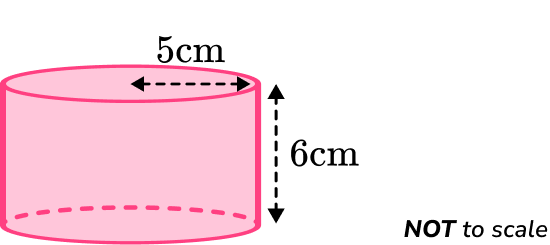471 \ cm^3157 \ cm^3565 \ cm^3188 \ cm^3The volume formula to calculate the volume of the cylinder is

\text{Volume} =\pi r^2h.

You need to substitute in the values r=5 and h=6.

\begin{aligned} \text{Volume} &=\pi r^2h \\\\ &= \pi \times 5^2 \times 6 \\\\ &=150\pi \\\\ &=471.23… \end{aligned}

The volume of the cylinder is 471 \ cm^3.

2. Calculate the volume of this pyramid.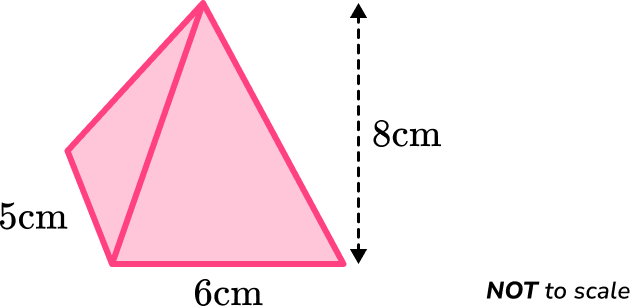240 \ cm^3200 \ cm^396 \ cm^380 \ cm^3The volume formula to calculate the volume of the pyramid is

\text{Volume} =\frac{1}{3}\times \text{area of base}\times \text{height}.

You need to find the area of the base of the pyramid.

A=5\times 6=30

\begin{aligned} \text{Volume} &=\frac{1}{3}\times \text{area of base}\times \text{height} \\\\ &= \frac{1}{3}\times 30\times 8 \\\\ &=80 \end{aligned}

The volume of the pyramid is 80 \ cm^3.

3. The volume of this 720 \ mm^3. Calculate the length of the missing side.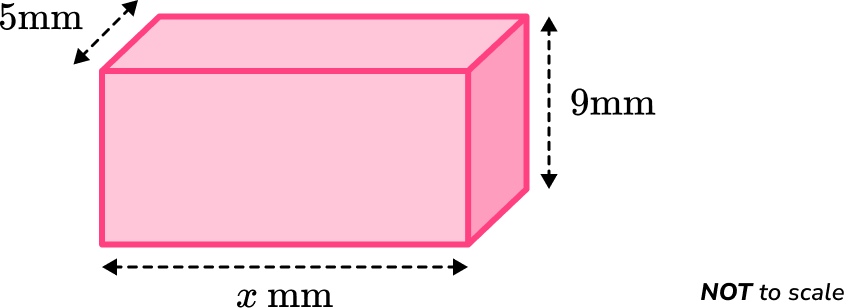16 \ mm16 \ cm15 \ mm15 \ cmThe volume formula to calculate the volume of the cuboid is

\text{Volume} =l\times w\times h.

You need to substitute in the values given, using x for the unknown length into the volume formula.

\begin{aligned} \text{Volume} &=l\times w\times h\\\\ 720&=x\times 5\times 9\\\\ 720&=x\times 45 \end{aligned}

The missing length is

x=720\div 45=16.

The missing length of the cuboid is 16 \ mm.

4. The volume of a cylinder is 160\pi \ cm^3. The height of the cylinder is 6.4 \ cm. Find the radius of the cylinder.

6 \ cm5 \ cm7 \ cm4 \ cmThe volume formula to calculate the volume of the cylinder is

\text{Volume} =\pi r^2h.

You need to substitute in the values given into the formula

\begin{aligned} \text{Volume} &=\pi r^2h\\\\ 160\pi&=\pi \times r^2\times 6.4 \end{aligned}

The workings for the missing length are

\begin{aligned} r^2&=\frac{160\pi}{\pi \times 6.4}\\\\ r^2&=25\\\\ r&=\sqrt{25}\\\\ r&=5 \end{aligned}

The radius of the cylinder is 5 \ cm.

5. Calculate the volume of the shape below.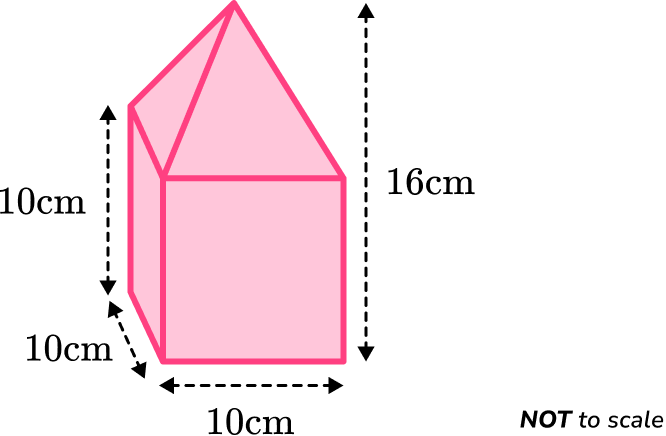1150 \ cm^31400 \ cm^31200 \ cm^32600 \ cm^3The volume of the cuboid is

\begin{aligned} \text{Volume}&=l\times w\times h\\\\ &=10\times 10\times 10\\\\ &=1000 \end{aligned}

Or you can take one side of the cube and cube it.

10^3=1000

The volume of the pyramid is

\begin{aligned} \text{Volume}&=\frac{1}{3}\times \text{area of base}\times \text{height}\\\\ &=\frac{1}{3}\times 10\times 10\times 6\\\\ &=200 \end{aligned}

The total volume can be found by adding the two volumes together.

\text{Total Volume}=1000+200=1200 \ cm^3

6. Calculate the volume of the shape below. Give your answer to 3 significant figures.516\pi \ cm^3660\pi \ cm^3684\pi \ cm^31116\pi \ cm^3The volume of the cylinder is

\begin{aligned} \text{Volume}&=\pi r^2h\\\\ &=\pi \times 6^2\times 12\\\\ &=432\pi \end{aligned}

The volume of the cone is

\begin{aligned} \text{Volume}&=\frac{1}{3}\pi r^2h\\\\ &=\frac{1}{3}\times \pi \times 6^2\times 7\\\\ &=84\pi \end{aligned}

The total volume can be found by adding the two volumes together.
\text{Total Volume}=432\pi+84\pi=516\pi \ cm^3

### Volume formula GCSE questions

1. Shown below is a prism.

The cross-sectional area is 16 \ cm^2.

The prism has length 11 \ cm.

Find the volume of the prism.

Include the units.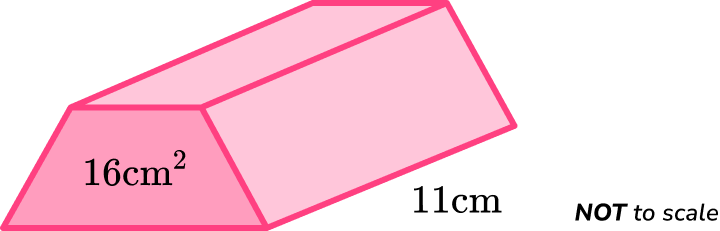(3 marks)

16 \times 11

(1)

176

(1)

cm^3

(1)

2. (a) Shown below is a cuboid.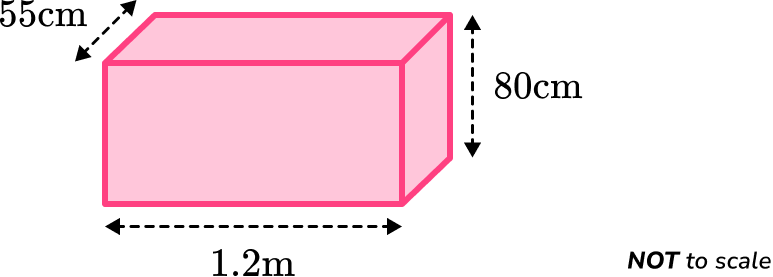The dimensions of the cuboid are 1.2 \ m, 80 \ cm and 55 \ cm.

Find the volume of the cuboid.

(b) The cuboid is filled with water.

You are given that 1 \ m^3=1000 \ litres.

Calculate the volume of water needed to fill the cuboid.

(5 marks)

(a)

80 \ cm =0.8 \ m or 55 \ cm = 0.55 \ m

(1)

1.2 \times 0.8 \times 0.55

(1)

0.528 \ m^3

(1)

(b)

0.528 \times 1000

(1)

528 \ litres

(1)

3. (a) The volume of a cylinder is 1350 \ cm^3.

The radius of the cylinder is 6.8 \ cm.

Calculate the height of the cylinder.

(b) The cylinder is made from pine wood.

Pine wood has a density of 373 \ kg/m^{3}.

Given that \text{Density}=\frac{\text{mass}}{\text{volume}} .

Calculate the mass of the cylinder.

(3 marks)

(a)

1350=\pi \times 6.8^{2} \times h

(1)

h=\frac{1350}{\pi \times 6.8^2}

(1)

9.29 \ cm

(1)

(b)

1350 \ cm^3=0.00135 \ m^3

(1)

373 \times 0.00135

(1)

0.50355 \ kg

(1)

4. The diagram shows a hollow pipe. It is made from a cylinder with diameter 10 \ m and height 16 \ m, with a cylindrical hole of diameter 8 \ m and height 16 \ m.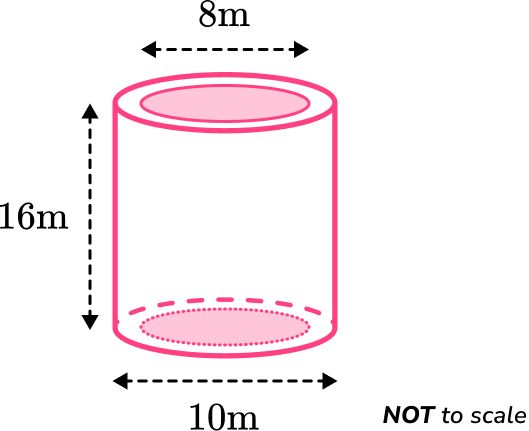Work out the volume of the hollow pipe.

(5 marks)

r_1=5 \ or \ r_2=4 \

(1)

V_1=\pi \times 5^2 \times 16 \ or \ V_2=\pi \times 4^2 \times 16

(1)

400\pi-256\pi

(1)

144\pi

(1)

m^3

(1)

5. The volume of a sphere is 3050 \ mm^3.

Calculate the diameter of the sphere.

(4 marks)

3050=\frac{4}{3} \times \pi \times r^3

(1)

r=\sqrt{\frac{3\times3050}{4\times \pi}}

(1)

d=2\times8.99643…

(1)

18.0 \ cm

(1)

## Learning checklist

You have now learned how to:

• Use a volume formula to calculate the volume of a 3D shape
• Use a volume formula to find a missing dimension of a shape given its volume
• Solve problems involving volume using a volume formula

## Still stuck?

Prepare your KS4 students for maths GCSEs success with Third Space Learning. Weekly online one to one GCSE maths revision lessons delivered by expert maths tutors.

Find out more about our GCSE maths tuition programme.